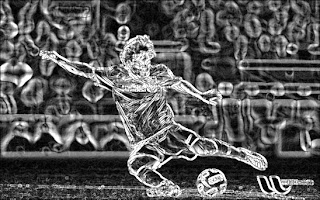## Sunday, June 3, 2012

### Image Derivatives and its Applications

Hi,

You can find image derivatives using cv2.Sobel() and cv2.Scharr() functions in OpenCV. There is a nice tutorial and explanation about this in OpenCV site, "Sobel Derivatives". You can find a Python adaptation here:  sobel.py

This post is written to show you some of those functions.This is the original image →First I applied Sobel derivatives in vertical and horizontal directions and blended them with equal weights, 0.5. Here is the result →Next, instead of blending, I directly added them. It gives you a much more bright result, just a fancy development, nothing special →Next, I applied Scharr instead of Sobel, and again blended them. Here is the result →

Scharr output is considered to be much more accurate.Next I applied Laplacian operator to the same image. It is sum of second derivatives in both the directions. If you use Sobel to find second derivative and take their sum, you get almost same result.

You can find tutorial about laplacian operator here: Laplace Operator. You can find corresponding Python implementation here : Python CodeFinally, there is Canny edge detector. Here is the result for canny edge detector for a low threshold value of 74. Original image and edge image is bitwise_and operated to make image a little colorful.

You can find tutorial about canny edge detector here : Canny Edge Detector. Its corresponding Python code is here : Python code

With Regards,
ARK

1.Hi Abid, I'm begginer in opencv and I don't knew opencv 2.0, how can I install it on linux ubuntu? thanks!

1.2.